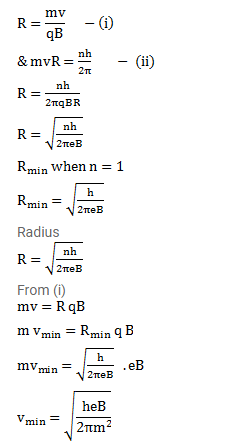# A uniform magnetic field B exists in a region.

Question:

A uniform magnetic field B exists in a region. An electron projected perpendicular to the field goes in a circle. Assuming Bohr's quantization rule for angular momentum, calculate

The smallest possible radius of the electron

The radius of the ${ }^{\text {nth }}$ orbit and

The minimum possible speed of the electron.

Solution: